## C# || How To Find Maximum Difference Between Node and Ancestor In Binary Tree Using C#The following is a module with functions which demonstrates how to find the maximum difference between node and ancestor in binary tree using C#.

1. Max Ancestor Diff – Problem Statement

Given the root of a binary tree, find the maximum value v for which there exist different nodes a and b where v = |a.val – b.val| and a is an ancestor of b.

A node a is an ancestor of b if either: any child of a is equal to b or any child of a is an ancestor of b.

Example 1: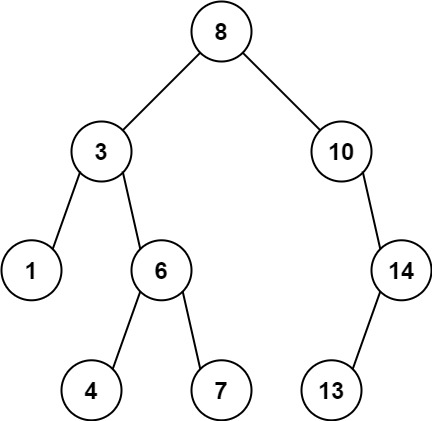``` Input: root = [8,3,10,1,6,null,14,null,null,4,7,13] Output: 7 Explanation: We have various ancestor-node differences, some of which are given below : |8 - 3| = 5 |3 - 7| = 4 |8 - 1| = 7 |10 - 13| = 3 Among all possible differences, the maximum value of 7 is obtained by |8 - 1| = 7. ```

Example 2: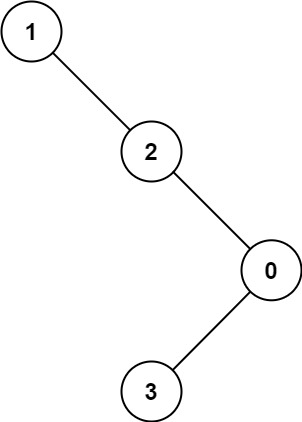``` Input: root = [1,null,2,null,0,3] Output: 3 ```

2. Max Ancestor Diff – Solution

The following is a solution which demonstrates how to find the maximum difference between node and ancestor in binary tree.

``` 2. Max Ancestor Diff - Solution C# // ============================================================================ // Author: Kenneth Perkins // Date: Feb 14, 2023 // Taken From: http://programmingnotes.org/ // File: Solution.cs // Description: Demonstrates how to find maximum difference node and ancestor // ============================================================================ /** * Definition for a binary tree node. * public class TreeNode { * public int val; * public TreeNode left; * public TreeNode right; * public TreeNode(int val=0, TreeNode left=null, TreeNode right=null) { * this.val = val; * this.left = left; * this.right = right; * } * } */ public class Solution { public int MaxAncestorDiff(TreeNode root) { if (root == null) { return 0; } return Traverse(root, root.val, root.val); } public int Traverse(TreeNode node, int curMax, int curMin) { // if encounter leaves, return the max-min along the path if (node == null) { return curMax - curMin; } // else, update max and min // and return the max of left and right subtrees curMax = Math.Max(curMax, node.val); curMin = Math.Min(curMin, node.val); int left = Traverse(node.left, curMax, curMin); int right = Traverse(node.right, curMax, curMin); return Math.Max(left, right); } }// http://programmingnotes.org/ 123456789101112131415161718192021222324252627282930313233343536373839404142 // ============================================================================//    Author: Kenneth Perkins//    Date:   Feb 14, 2023//    Taken From: http://programmingnotes.org///    File:  Solution.cs//    Description: Demonstrates how to find maximum difference node and ancestor// ============================================================================/** * Definition for a binary tree node. * public class TreeNode { *     public int val; *     public TreeNode left; *     public TreeNode right; *     public TreeNode(int val=0, TreeNode left=null, TreeNode right=null) { *         this.val = val; *         this.left = left; *         this.right = right; *     } * } */public class Solution {    public int MaxAncestorDiff(TreeNode root) {        if (root == null) {            return 0;        }        return Traverse(root, root.val, root.val);    }     public int Traverse(TreeNode node, int curMax, int curMin) {        // if encounter leaves, return the max-min along the path        if (node == null) {            return curMax - curMin;        }        // else, update max and min        // and return the max of left and right subtrees        curMax = Math.Max(curMax, node.val);        curMin = Math.Min(curMin, node.val);        int left = Traverse(node.left, curMax, curMin);        int right = Traverse(node.right, curMax, curMin);        return Math.Max(left, right);    }}// http://programmingnotes.org/ ```

QUICK NOTES:
The highlighted lines are sections of interest to look out for.

The code is heavily commented, so no further insight is necessary. If you have any questions, feel free to leave a comment below.

Once compiled, you should get this as your output for the example cases:

``` 7 3 ```

## C# || Two Sum IV – How To Get Two Numbers In Binary Search Tree Equal To Target Value Using C#The following is a module with functions which demonstrates how to get two numbers in a binary search tree equal to target value using C#.

1. Find Target – Problem Statement

Given the root of a Binary Search Tree and a target number k, return true if there exist two elements in the BST such that their sum is equal to the given target.

Example 1:``` Input: root = [5,3,6,2,4,null,7], k = 9 Output: true ```

Example 2: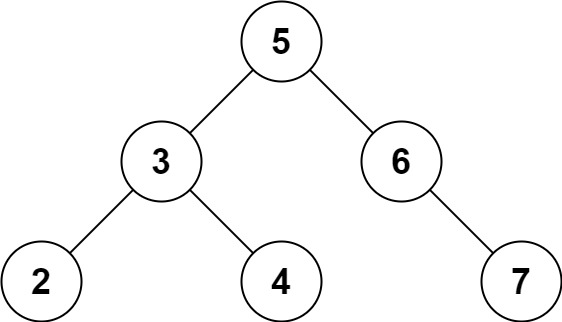``` Input: root = [5,3,6,2,4,null,7], k = 28 Output: false ```

2. Find Target – Solution

The following are two solutions which demonstrates how to get two numbers in a binary search tree equal to target value.

Both solutions use a set to keep track of the items already seen.

Each time a new node is encountered, we subtract the target value from the current node value. If the difference amount from subtracting the two numbers exists in the set, a 2 sum combination exists in the tree

1. Recursive

The following solution uses Depth First Search when looking for the target value.

``` 2. Find Target - Solution - Recursive C# // ============================================================================ // Author: Kenneth Perkins // Date: Oct 9, 2022 // Taken From: http://programmingnotes.org/ // File: Solution.cs // Description: Demonstrates how to get two numbers equal to target value // ============================================================================ public class Solution { public bool FindTarget(TreeNode root, int k) { return Traverse(root, k, new HashSet<int>()); } private bool Traverse(TreeNode node, int k, HashSet<int> seen) { if (node == null) { return false; } // Get remaining value var remaining = k - node.val; // Check if remaining value has been seen if (seen.Contains(remaining)) { return true; } // Add current node value to items seen seen.Add(node.val); // Keep traversing left and right looking for 2 sum return Traverse(node.left, k, seen) || Traverse(node.right, k, seen); } }// http://programmingnotes.org/ 123456789101112131415161718192021222324252627282930 // ============================================================================//    Author: Kenneth Perkins//    Date:   Oct 9, 2022//    Taken From: http://programmingnotes.org///    File:  Solution.cs//    Description: Demonstrates how to get two numbers equal to target value// ============================================================================public class Solution {    public bool FindTarget(TreeNode root, int k) {        return Traverse(root, k, new HashSet<int>());    }     private bool Traverse(TreeNode node, int k, HashSet<int> seen) {        if (node == null) {            return false;        }        // Get remaining value        var remaining = k - node.val;         // Check if remaining value has been seen        if (seen.Contains(remaining)) {            return true;        }        // Add current node value to items seen        seen.Add(node.val);         // Keep traversing left and right looking for 2 sum        return Traverse(node.left, k, seen) || Traverse(node.right, k, seen);    }}// http://programmingnotes.org/ ```

2. Iterative

The following solution uses Breadth First Search when looking for the target value.

``` 2. Find Target - Solution - Iterative C# // ============================================================================ // Author: Kenneth Perkins // Date: Oct 9, 2022 // Taken From: http://programmingnotes.org/ // File: Solution.cs // Description: Demonstrates how to get two numbers equal to target value // ============================================================================ public class Solution { public bool FindTarget(TreeNode root, int k) { if (root == null) { return false; } // Declare stack var stack = new Stack<TreeNode>(); // Keep track of values seen var seen = new HashSet<int>(); // Add root to stack stack.Push(root); // Loop through items on the stack while (stack.Count > 0) { // Get current node var node = stack.Pop(); // Get remaining value var remaining = k - node.val; // Check if remaining value has been seen if (seen.Contains(remaining)) { return true; } else { // Keep traversing left and right looking for 2 sum if (node.left != null) { stack.Push(node.left); } if (node.right != null) { stack.Push(node.right); } } // Add current node value to items seen seen.Add(node.val); } return false; } }// http://programmingnotes.org/ 1234567891011121314151617181920212223242526272829303132333435363738394041424344454647 // ============================================================================//    Author: Kenneth Perkins//    Date:   Oct 9, 2022//    Taken From: http://programmingnotes.org///    File:  Solution.cs//    Description: Demonstrates how to get two numbers equal to target value// ============================================================================public class Solution {    public bool FindTarget(TreeNode root, int k) {        if (root == null) {            return false;        }        // Declare stack        var stack = new Stack<TreeNode>();         // Keep track of values seen        var seen = new HashSet<int>();         // Add root to stack        stack.Push(root);         // Loop through items on the stack        while (stack.Count > 0) {            // Get current node            var node = stack.Pop();             // Get remaining value            var remaining = k - node.val;             // Check if remaining value has been seen            if (seen.Contains(remaining)) {                return true;            } else {                // Keep traversing left and right looking for 2 sum                if (node.left != null) {                    stack.Push(node.left);                }                if (node.right != null) {                    stack.Push(node.right);                }            }            // Add current node value to items seen            seen.Add(node.val);        }        return false;    }}// http://programmingnotes.org/ ```

QUICK NOTES:
The highlighted lines are sections of interest to look out for.

The code is heavily commented, so no further insight is necessary. If you have any questions, feel free to leave a comment below.

Once compiled, you should get this as your output for the example cases:

``` true false ```

## C# || How To Traverse N-ary Tree Level Order Using C#The following is a module with functions which demonstrates how to traverse a N-ary Tree level order using C#.

1. Level Order – Problem Statement

Given an n-ary tree, return the level order traversal of its nodes’ values.

Nary-Tree input serialization is represented in their level order traversal, each group of children is separated by the null value (See examples).

Example 1: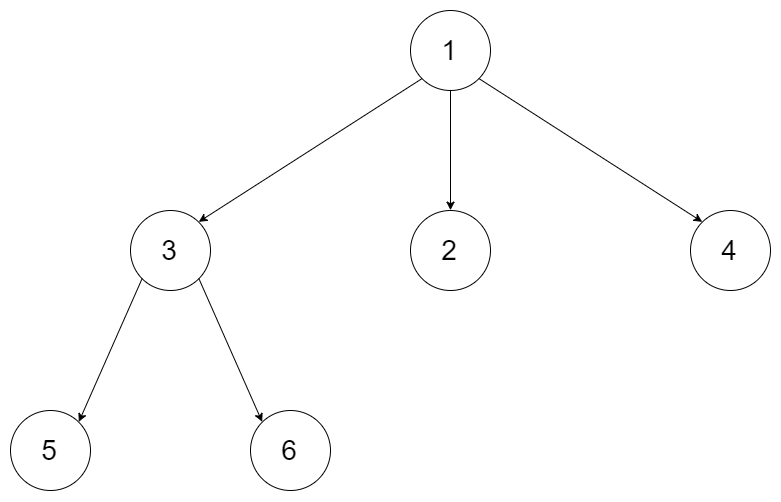``` Input: root = [1,null,3,2,4,null,5,6] Output: [,[3,2,4],[5,6]] ```

Example 2: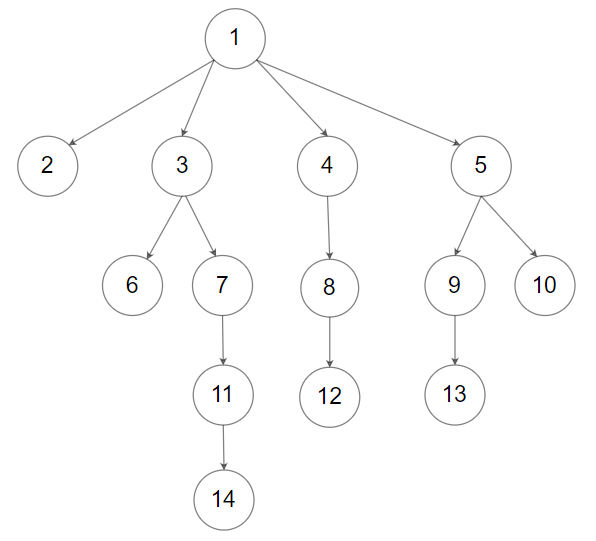``` Input: root = [1,null,2,3,4,5,null,null,6,7,null,8,null,9,10,null,null,11,null,12,null,13,null,null,14] Output: [,[2,3,4,5],[6,7,8,9,10],[11,12,13],] ```

2. Level Order – Solution

The following is a solution which demonstrates how to traverse a N-ary Tree level order.

This solution uses Breadth First Search to explore items at each level.

``` 2. Level Order - Solution C# // ============================================================================ // Author: Kenneth Perkins // Date: Sep 5, 2022 // Taken From: http://programmingnotes.org/ // File: Solution.cs // Description: Demonstrates how to traverse a N-ary Tree Level Order // ============================================================================ /* // Definition for a Node. public class Node { public int val; public IList<Node> children; public Node() {} public Node(int _val) { val = _val; } public Node(int _val, IList<Node> _children) { val = _val; children = _children; } } */ public class Solution { public IList<IList<int>> LevelOrder(Node root) { var result = new List<IList<int>>(); if (root == null) { return result; } var queue = new Queue<Node>(); queue.Enqueue(root); while (queue.Count > 0) { var currentLevel = new List<int>(); for (int count = queue.Count - 1; count >= 0 ; --count) { var currentNode = queue.Dequeue(); currentLevel.Add(currentNode.val); foreach (var child in currentNode.children) { queue.Enqueue(child); } } result.Add(currentLevel); } return result; } }// http://programmingnotes.org/ 1234567891011121314151617181920212223242526272829303132333435363738394041424344454647484950 // ============================================================================//    Author: Kenneth Perkins//    Date:   Sep 5, 2022//    Taken From: http://programmingnotes.org///    File:  Solution.cs//    Description: Demonstrates how to traverse a N-ary Tree Level Order// ============================================================================/*// Definition for a Node.public class Node {    public int val;    public IList<Node> children;     public Node() {}     public Node(int _val) {        val = _val;    }     public Node(int _val, IList<Node> _children) {        val = _val;        children = _children;    }}*/public class Solution {    public IList<IList<int>> LevelOrder(Node root) {        var result = new List<IList<int>>();        if (root == null) {           return result;        }         var queue = new Queue<Node>();        queue.Enqueue(root);         while (queue.Count > 0) {            var currentLevel = new List<int>();            for (int count = queue.Count - 1; count >= 0 ; --count) {                var currentNode = queue.Dequeue();                currentLevel.Add(currentNode.val);                foreach (var child in currentNode.children) {                    queue.Enqueue(child);                }            }            result.Add(currentLevel);        }         return result;    }}// http://programmingnotes.org/ ```

QUICK NOTES:
The highlighted lines are sections of interest to look out for.

The code is heavily commented, so no further insight is necessary. If you have any questions, feel free to leave a comment below.

Once compiled, you should get this as your output for the example cases:

``` [,[3,2,4],[5,6]] [,[2,3,4,5],[6,7,8,9,10],[11,12,13],] ```

## C# || How To Validate A Binary Search Tree Using C#The following is a module with functions which demonstrates how to validate a binary search tree using C#.

1. Is Valid BST – Problem Statement

Given the root of a binary tree, determine if it is a valid binary search tree (BST).

A valid BST is defined as follows:

• The left subtree of a node contains only nodes with keys less than the node’s key.
• The right subtree of a node contains only nodes with keys greater than the node’s key.
• Both the left and right subtrees must also be binary search trees.

Example 1: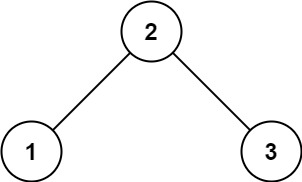``` Input: root = [2,1,3] Output: true ```

Example 2:``` Input: root = [5,1,4,null,null,3,6] Output: false Explanation: The root node's value is 5 but its right child's value is 4. ```

2. Is Valid BST – Solution

The following is a solution which demonstrates how to validate a binary search tree.

``` 2. Is Valid BST - Solution C# // ============================================================================ // Author: Kenneth Perkins // Date: Aug 12, 2022 // Taken From: http://programmingnotes.org/ // File: Solution.cs // Description: Demonstrates how to validate a BST // ============================================================================ /** * Definition for a binary tree node. * public class TreeNode { * public int val; * public TreeNode left; * public TreeNode right; * public TreeNode(int val=0, TreeNode left=null, TreeNode right=null) { * this.val = val; * this.left = left; * this.right = right; * } * } */ public class Solution { public bool IsValidBST(TreeNode root) { return Traverse(root, null, null); } private bool Traverse(TreeNode node, TreeNode min, TreeNode max) { if (node == null) { return true; } if (max != null && node.val >= max.val) { return false; } if (min != null && node.val <= min.val) { return false; } return Traverse(node.left, min, node) && Traverse(node.right, node, max); } }// http://programmingnotes.org/ 123456789101112131415161718192021222324252627282930313233343536373839 // ============================================================================//    Author: Kenneth Perkins//    Date:   Aug 12, 2022//    Taken From: http://programmingnotes.org///    File:  Solution.cs//    Description: Demonstrates how to validate a BST// ============================================================================/** * Definition for a binary tree node. * public class TreeNode { *     public int val; *     public TreeNode left; *     public TreeNode right; *     public TreeNode(int val=0, TreeNode left=null, TreeNode right=null) { *         this.val = val; *         this.left = left; *         this.right = right; *     } * } */public class Solution {    public bool IsValidBST(TreeNode root) {        return Traverse(root, null, null);    }     private bool Traverse(TreeNode node, TreeNode min, TreeNode max) {        if (node == null) {            return true;        }        if (max != null && node.val >= max.val) {            return false;        }        if (min != null && node.val <= min.val) {            return false;        }        return Traverse(node.left, min, node)            && Traverse(node.right, node, max);    }}// http://programmingnotes.org/ ```

QUICK NOTES:
The highlighted lines are sections of interest to look out for.

The code is heavily commented, so no further insight is necessary. If you have any questions, feel free to leave a comment below.

Once compiled, you should get this as your output for the example cases:

``` true false ```

## C# || Binary Tree Right Side View – How To Get Nodes Ordered Top To Bottom C#The following is a module with functions which demonstrates how to get nodes in a binary tree ordered from top to bottom using C#.

1. Right Side View – Problem Statement

Given the root of a binary tree, imagine yourself standing on the right side of it, return the values of the nodes you can see ordered from top to bottom.

Example 1: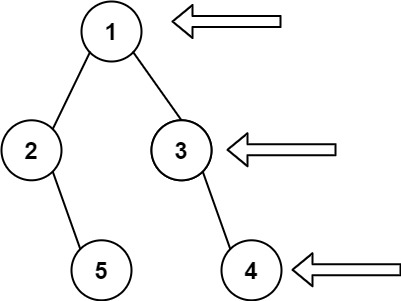``` Input: root = [1,2,3,null,5,null,4] Output: [1,3,4] ```

Example 2:

``` Input: root = [1,null,3] Output: [1,3] ```

Example 3:

``` Input: root = [] Output: [] ```

2. Right Side View – Solution

The following is a solution which demonstrates how to get right side nodes ordered from top to bottom.

This solution uses Depth First Search level order traversal to explore items at each level, and then adds the last node on every layer.

``` 2. Right Side View - Solution C# // ============================================================================ // Author: Kenneth Perkins // Date: Jul 10, 2022 // Taken From: http://programmingnotes.org/ // File: Solution.cs // Description: Demonstrates how to get nodes ordered from top to bottom // ============================================================================ /** * Definition for a binary tree node. * public class TreeNode { * public int val; * public TreeNode left; * public TreeNode right; * public TreeNode(int val=0, TreeNode left=null, TreeNode right=null) { * this.val = val; * this.left = left; * this.right = right; * } * } */ public class Solution { public IList<int> RightSideView(TreeNode root) { var result = new List<int>(); Traverse(root, 0, result); return result; } public void Traverse(TreeNode node, int currentDepth, List<int> result) { if (node == null) { return; } if (currentDepth == result.Count) { result.Add(node.val); } Traverse(node.right, currentDepth + 1, result); Traverse(node.left, currentDepth + 1, result); } }// http://programmingnotes.org/ 1234567891011121314151617181920212223242526272829303132333435363738 // ============================================================================//    Author: Kenneth Perkins//    Date:   Jul 10, 2022//    Taken From: http://programmingnotes.org///    File:  Solution.cs//    Description: Demonstrates how to get nodes ordered from top to bottom// ============================================================================/** * Definition for a binary tree node. * public class TreeNode { *     public int val; *     public TreeNode left; *     public TreeNode right; *     public TreeNode(int val=0, TreeNode left=null, TreeNode right=null) { *         this.val = val; *         this.left = left; *         this.right = right; *     } * } */public class Solution {    public IList<int> RightSideView(TreeNode root) {        var result = new List<int>();        Traverse(root, 0, result);        return result;    }     public void Traverse(TreeNode node, int currentDepth, List<int> result) {        if (node == null) {            return;        }        if (currentDepth == result.Count) {            result.Add(node.val);        }        Traverse(node.right, currentDepth + 1, result);        Traverse(node.left, currentDepth + 1, result);    }}// http://programmingnotes.org/ ```

QUICK NOTES:
The highlighted lines are sections of interest to look out for.

The code is heavily commented, so no further insight is necessary. If you have any questions, feel free to leave a comment below.

Once compiled, you should get this as your output for the example cases:

``` [1,3,4] [1,3] [] ```

## C# || How To Get Total Sum Root To Leaf Binary Numbers In Binary Tree Using C#The following is a module with functions which demonstrates how to get the total sum root to leaf binary numbers in a binary tree using C#.

1. Sum Root To Leaf – Problem Statement

You are given the root of a binary tree where each node has a value 0 or 1. Each root-to-leaf path represents a binary number starting with the most significant bit.

• For example, if the path is 0 -> 1 -> 1 -> 0 -> 1, then this could represent 01101 in binary, which is 13.

For all leaves in the tree, consider the numbers represented by the path from the root to that leaf. Return the sum of these numbers.

The test cases are generated so that the answer fits in a 32-bits integer.

A leaf node is a node with no children.

Example 1: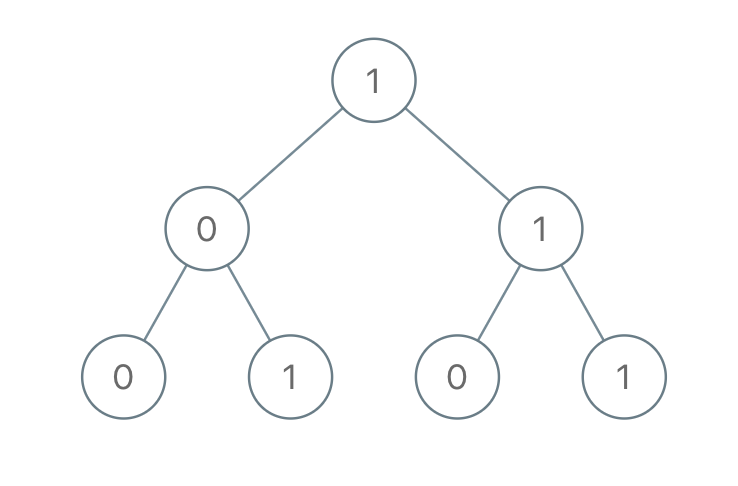``` Input: root = [1,0,1,0,1,0,1] Output: 22 Explanation: (100) + (101) + (110) + (111) = 4 + 5 + 6 + 7 = 22 ```

Example 2:

``` Input: root =  Output: 0 ```

2. Sum Root To Leaf – Solution

The following is a solution which demonstrates how to get the total sum root to leaf binary numbers in a binary tree.

This solution uses Depth First Search to explore items at each level.

``` 2. Sum Root To Leaf - Solution C# // ============================================================================ // Author: Kenneth Perkins // Date: Jan 10, 2022 // Taken From: http://programmingnotes.org/ // File: Solution.cs // Description: Demonstrates how to get sum root to leaf binary numbers // ============================================================================ /** * Definition for a binary tree node. * public class TreeNode { * public int val; * public TreeNode left; * public TreeNode right; * public TreeNode(int val=0, TreeNode left=null, TreeNode right=null) { * this.val = val; * this.left = left; * this.right = right; * } * } */ public class Solution { public int SumRootToLeaf(TreeNode root) { return Traverse(root, 0); } private int Traverse(TreeNode node, int currentSum) { if (node == null) { return 0; } // Calculate current sum currentSum = (currentSum * 2) + node.val; // We have reached a leaf node if (node.left == null && node.right == null) { return currentSum; } // Keep traversing left and right calculating the sum return Traverse(node.left, currentSum) + Traverse(node.right, currentSum); } }// http://programmingnotes.org/ 123456789101112131415161718192021222324252627282930313233343536373839 // ============================================================================//    Author: Kenneth Perkins//    Date:   Jan 10, 2022//    Taken From: http://programmingnotes.org///    File:  Solution.cs//    Description: Demonstrates how to get sum root to leaf binary numbers// ============================================================================/** * Definition for a binary tree node. * public class TreeNode { *     public int val; *     public TreeNode left; *     public TreeNode right; *     public TreeNode(int val=0, TreeNode left=null, TreeNode right=null) { *         this.val = val; *         this.left = left; *         this.right = right; *     } * } */public class Solution {    public int SumRootToLeaf(TreeNode root) {        return Traverse(root, 0);    }    private int Traverse(TreeNode node, int currentSum) {        if (node == null) {            return 0;        }        // Calculate current sum        currentSum = (currentSum * 2) + node.val;         // We have reached a leaf node        if (node.left == null && node.right == null) {            return currentSum;        }        // Keep traversing left and right calculating the sum        return Traverse(node.left, currentSum) + Traverse(node.right, currentSum);    }}// http://programmingnotes.org/ ```

QUICK NOTES:
The highlighted lines are sections of interest to look out for.

The code is heavily commented, so no further insight is necessary. If you have any questions, feel free to leave a comment below.

Once compiled, you should get this as your output for the example cases:

``` 22 0 ```

## C# || How To Construct Binary Tree From Preorder And Inorder Traversal Using C#The following is a module with functions which demonstrates how to construct a binary tree from pre order and in order traversal using C#.

1. Build Tree – Problem Statement

Given two integer arrays preorder and inorder where preorder is the preorder traversal of a binary tree and inorder is the inorder traversal of the same tree, construct and return the binary tree.

Example 1: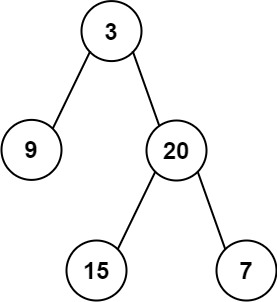``` Input: preorder = [3,9,20,15,7], inorder = [9,3,15,20,7] Output: [3,9,20,null,null,15,7] ```

Example 2:

``` Input: preorder = [-1], inorder = [-1] Output: [-1] ```

2. Build Tree – Solution

The following is a solution which demonstrates how to construct a binary tree from pre order and in order traversal.

``` 2. Build Tree - Solution C# // ============================================================================ // Author: Kenneth Perkins // Date: Nov 20, 2021 // Taken From: http://programmingnotes.org/ // File: Solution.cs // Description: Demonstrates how to build binary tree from pre order and in order // ============================================================================ /** * Definition for a binary tree node. * public class TreeNode { * public int val; * public TreeNode left; * public TreeNode right; * public TreeNode(int val=0, TreeNode left=null, TreeNode right=null) { * this.val = val; * this.left = left; * this.right = right; * } * } */ public class Solution { private int preorderIndex; private Dictionary<int, int> inorderIndexMap; public TreeNode BuildTree(int[] preorder, int[] inorder) { preorderIndex = 0; // Build a hashmap to store value -> its index relations inorderIndexMap = new Dictionary<int, int>(); for (int index = 0; index < inorder.Length; ++index) { inorderIndexMap[inorder[index]] = index; } return ArrayToTree(preorder, 0, preorder.Length - 1); } private TreeNode ArrayToTree(int[] preorder, int start, int end) { // If there are no elements to construct the tree if (start > end) { return null; } // Select the preorder_index element as the root and increment it var rootValue = preorder[preorderIndex++]; var root = new TreeNode(rootValue); // Get current positon var curPos = inorderIndexMap[rootValue]; // Build left and right subtree // excluding inorderIndexMap[rootValue] element because it's the root root.left = ArrayToTree(preorder, start, curPos - 1); root.right = ArrayToTree(preorder, curPos + 1, end); return root; } }// http://programmingnotes.org/ 12345678910111213141516171819202122232425262728293031323334353637383940414243444546474849505152535455 // ============================================================================//    Author: Kenneth Perkins//    Date:   Nov 20, 2021//    Taken From: http://programmingnotes.org///    File:  Solution.cs//    Description: Demonstrates how to build binary tree from pre order and in order// ============================================================================/** * Definition for a binary tree node. * public class TreeNode { *     public int val; *     public TreeNode left; *     public TreeNode right; *     public TreeNode(int val=0, TreeNode left=null, TreeNode right=null) { *         this.val = val; *         this.left = left; *         this.right = right; *     } * } */public class Solution {    private int preorderIndex;    private Dictionary<int, int> inorderIndexMap;     public TreeNode BuildTree(int[] preorder, int[] inorder) {        preorderIndex = 0;         // Build a hashmap to store value -> its index relations        inorderIndexMap = new Dictionary<int, int>();        for (int index = 0; index < inorder.Length; ++index) {            inorderIndexMap[inorder[index]] = index;        }        return ArrayToTree(preorder, 0, preorder.Length - 1);    }     private TreeNode ArrayToTree(int[] preorder, int start, int end) {        // If there are no elements to construct the tree        if (start > end) {            return null;        }         // Select the preorder_index element as the root and increment it        var rootValue = preorder[preorderIndex++];        var root = new TreeNode(rootValue);         // Get current positon        var curPos = inorderIndexMap[rootValue];         // Build left and right subtree        // excluding inorderIndexMap[rootValue] element because it's the root        root.left = ArrayToTree(preorder, start, curPos - 1);        root.right = ArrayToTree(preorder, curPos + 1, end);        return root;    }}// http://programmingnotes.org/ ```

QUICK NOTES:
The highlighted lines are sections of interest to look out for.

The code is heavily commented, so no further insight is necessary. If you have any questions, feel free to leave a comment below.

Once compiled, you should get this as your output for the example cases:

``` [3,9,20,null,null,15,7] [-1] ```

## C# || How To Construct Binary Tree From Inorder And Postorder Traversal Using C#The following is a module with functions which demonstrates how to construct a binary tree from in order and post order traversal using C#.

1. Build Tree – Problem Statement

Given two integer arrays inorder and postorder where inorder is the inorder traversal of a binary tree and postorder is the postorder traversal of the same tree, construct and return the binary tree.

Example 1:``` Input: inorder = [9,3,15,20,7], postorder = [9,15,7,20,3] Output: [3,9,20,null,null,15,7] ```

Example 2:

``` Input: inorder = [-1], postorder = [-1] Output: [-1] ```

2. Build Tree – Solution

The following is a solution which demonstrates how to construct a binary tree from in order and post order traversal.

``` 2. Build Tree - Solution C# // ============================================================================ // Author: Kenneth Perkins // Date: Nov 20, 2021 // Taken From: http://programmingnotes.org/ // File: Solution.cs // Description: Demonstrates how to build binary tree from in order and post order // ============================================================================ /** * Definition for a binary tree node. * public class TreeNode { * public int val; * public TreeNode left; * public TreeNode right; * public TreeNode(int val=0, TreeNode left=null, TreeNode right=null) { * this.val = val; * this.left = left; * this.right = right; * } * } */ public class Solution { private int postorderIndex; private Dictionary<int, int> inorderIndexMap; public TreeNode BuildTree(int[] inorder, int[] postorder) { postorderIndex = postorder.Length - 1; // Build a map to store value & its index relation inorderIndexMap = new Dictionary<int, int>(); for (int index = 0; index < inorder.Length; ++index) { inorderIndexMap[inorder[index]] = index; } return ArrayToTree(postorder, 0, postorder.Length - 1); } private TreeNode ArrayToTree(int[] postorder, int start, int end) { // If there are no elements to construct the tree if (start > end) { return null; } // Select the postorder element as the root and decrement it var rootValue = postorder[postorderIndex--]; var root = new TreeNode(rootValue); // Get current positon var curPos = inorderIndexMap[rootValue]; // Build left and right subtree // excluding inorderIndexMap[rootValue] element because it's the root root.right = ArrayToTree(postorder, curPos + 1, end); root.left = ArrayToTree(postorder, start, curPos - 1); return root; } }// http://programmingnotes.org/ 12345678910111213141516171819202122232425262728293031323334353637383940414243444546474849505152535455 // ============================================================================//    Author: Kenneth Perkins//    Date:   Nov 20, 2021//    Taken From: http://programmingnotes.org///    File:  Solution.cs//    Description: Demonstrates how to build binary tree from in order and post order// ============================================================================/** * Definition for a binary tree node. * public class TreeNode { *     public int val; *     public TreeNode left; *     public TreeNode right; *     public TreeNode(int val=0, TreeNode left=null, TreeNode right=null) { *         this.val = val; *         this.left = left; *         this.right = right; *     } * } */public class Solution {    private int postorderIndex;    private Dictionary<int, int> inorderIndexMap;     public TreeNode BuildTree(int[] inorder, int[] postorder) {        postorderIndex = postorder.Length - 1;         // Build a map to store value & its index relation        inorderIndexMap = new Dictionary<int, int>();        for (int index = 0; index < inorder.Length; ++index) {            inorderIndexMap[inorder[index]] = index;        }        return ArrayToTree(postorder, 0, postorder.Length - 1);    }     private TreeNode ArrayToTree(int[] postorder, int start, int end) {        // If there are no elements to construct the tree        if (start > end) {            return null;        }         // Select the postorder element as the root and decrement it        var rootValue = postorder[postorderIndex--];        var root = new TreeNode(rootValue);         // Get current positon        var curPos = inorderIndexMap[rootValue];         // Build left and right subtree        // excluding inorderIndexMap[rootValue] element because it's the root        root.right = ArrayToTree(postorder, curPos + 1, end);        root.left = ArrayToTree(postorder, start, curPos - 1);        return root;    }}// http://programmingnotes.org/ ```

QUICK NOTES:
The highlighted lines are sections of interest to look out for.

The code is heavily commented, so no further insight is necessary. If you have any questions, feel free to leave a comment below.

Once compiled, you should get this as your output for the example cases:

``` [3,9,20,null,null,15,7] [-1] ```

## C# || How To Get Total Sum Of Left Leaves In Binary Tree Using C#The following is a module with functions which demonstrates how to get the total sum of left leaves in a binary tree using C#.

1. Sum Of Left Leaves – Problem Statement

Given the root of a binary tree, return the sum of all left leaves.

A leaf node is a node with no children.

Example 1:``` Input: root = [3,9,20,null,null,15,7] Output: 24 Explanation: There are two left leaves in the binary tree, with values 9 and 15 respectively. ```

Example 2:

``` Input: root =  Output: 0 ```

2. Sum Of Left Leaves – Solution

The following are two solutions which demonstrates how to get the total sum of left leaves in a binary tree.

Both solutions use Depth First Search to explore items at each level.

In both solutions, we traverse the left and right side of the tree, keeping track of which node is the ‘left’ node.

When a leaf is encountered and its a node on the left side, we increment the final result with the value of the node on the left side.

Recursive

``` 2. Sum Of Left Leaves - Recursive - Solution C# // ============================================================================ // Author: Kenneth Perkins // Date: Nov 3, 2021 // Taken From: http://programmingnotes.org/ // File: Solution.cs // Description: Demonstrates how to get the sum of left leaves // ============================================================================ /** * Definition for a binary tree node. * public class TreeNode { * public int val; * public TreeNode left; * public TreeNode right; * public TreeNode(int val=0, TreeNode left=null, TreeNode right=null) { * this.val = val; * this.left = left; * this.right = right; * } * } */ public class Solution { public int SumOfLeftLeaves(TreeNode root) { return Traverse(root, false); } private int Traverse(TreeNode node, bool isLeft) { if (node == null) { return 0; } // We have reached a leaf node if (node.left == null && node.right == null) { return isLeft ? node.val : 0; } // Keep traversing left and right looking for left leaves return Traverse(node.left, true) + Traverse(node.right, false); } }// http://programmingnotes.org/ 12345678910111213141516171819202122232425262728293031323334353637 // ============================================================================//    Author: Kenneth Perkins//    Date:   Nov 3, 2021//    Taken From: http://programmingnotes.org///    File:  Solution.cs//    Description: Demonstrates how to get the sum of left leaves// ============================================================================/** * Definition for a binary tree node. * public class TreeNode { *     public int val; *     public TreeNode left; *     public TreeNode right; *     public TreeNode(int val=0, TreeNode left=null, TreeNode right=null) { *         this.val = val; *         this.left = left; *         this.right = right; *     } * } */public class Solution {    public int SumOfLeftLeaves(TreeNode root) {        return Traverse(root, false);    }     private int Traverse(TreeNode node, bool isLeft) {        if (node == null) {            return 0;        }        // We have reached a leaf node        if (node.left == null && node.right == null) {            return isLeft ? node.val : 0;        }        // Keep traversing left and right looking for left leaves        return Traverse(node.left, true) + Traverse(node.right, false);    }}// http://programmingnotes.org/ ```

Iterative

``` 2. Sum Of Left Leaves - Iterative - Solution C# // ============================================================================ // Author: Kenneth Perkins // Date: Nov 3, 2021 // Taken From: http://programmingnotes.org/ // File: Solution.cs // Description: Demonstrates how to get the sum of left leaves // ============================================================================ /** * Definition for a binary tree node. * public class TreeNode { * public int val; * public TreeNode left; * public TreeNode right; * public TreeNode(int val=0, TreeNode left=null, TreeNode right=null) { * this.val = val; * this.left = left; * this.right = right; * } * } */ public class Solution { public int SumOfLeftLeaves(TreeNode root) { if (root == null) { return 0; } // Declare stack var stack = new Stack<KeyValuePair<TreeNode, bool>>(); var result = 0; // Add root to stack stack.Push(new KeyValuePair<TreeNode, bool>(root, false)); // Loop through items on the stack while (stack.Count > 0) { // Get current node and value indicating if its a left node var current = stack.Pop(); var node = current.Key; var isLeft = current.Value; // We have reached a leaf node if (node.left == null && node.right == null) { result += isLeft ? node.val : 0; } else { // Keep traversing left and right looking for left leaves if (node.left != null) { stack.Push(new KeyValuePair<TreeNode, bool>(node.left, true)); } if (node.right != null) { stack.Push(new KeyValuePair<TreeNode, bool>(node.right, false)); } } } return result; } }// http://programmingnotes.org/ 1234567891011121314151617181920212223242526272829303132333435363738394041424344454647484950515253545556 // ============================================================================//    Author: Kenneth Perkins//    Date:   Nov 3, 2021//    Taken From: http://programmingnotes.org///    File:  Solution.cs//    Description: Demonstrates how to get the sum of left leaves// ============================================================================/** * Definition for a binary tree node. * public class TreeNode { *     public int val; *     public TreeNode left; *     public TreeNode right; *     public TreeNode(int val=0, TreeNode left=null, TreeNode right=null) { *         this.val = val; *         this.left = left; *         this.right = right; *     } * } */public class Solution {    public int SumOfLeftLeaves(TreeNode root) {        if (root == null) {            return 0;        }        // Declare stack        var stack = new Stack<KeyValuePair<TreeNode, bool>>();        var result = 0;         // Add root to stack        stack.Push(new KeyValuePair<TreeNode, bool>(root, false));         // Loop through items on the stack        while (stack.Count > 0) {            // Get current node and value indicating if its a left node            var current = stack.Pop();            var node = current.Key;            var isLeft = current.Value;             // We have reached a leaf node            if (node.left == null && node.right == null) {                result += isLeft ? node.val : 0;            } else {                // Keep traversing left and right looking for left leaves                if (node.left != null) {                    stack.Push(new KeyValuePair<TreeNode, bool>(node.left, true));                }                if (node.right != null) {                    stack.Push(new KeyValuePair<TreeNode, bool>(node.right, false));                }            }        }         return result;    }}// http://programmingnotes.org/ ```

QUICK NOTES:
The highlighted lines are sections of interest to look out for.

The code is heavily commented, so no further insight is necessary. If you have any questions, feel free to leave a comment below.

Once compiled, you should get this as your output for the example cases:

``` 24 0 ```

## C# || How To Get Total Sum Root To Leaf Numbers In Binary Tree Using C#The following is a module with functions which demonstrates how to get the total sum root to leaf numbers in a binary tree using C#.

1. Sum Numbers – Problem Statement

You are given the root of a binary tree containing digits from 0 to 9 only.

Each root-to-leaf path in the tree represents a number.

• For example, the root-to-leaf path 1 -> 2 -> 3 represents the number 123.

Return the total sum of all root-to-leaf numbers. Test cases are generated so that the answer will fit in a 32-bit integer.

A leaf node is a node with no children.

Example 1: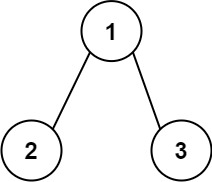``` Input: root = [1,2,3] Output: 25 Explanation: The root-to-leaf path 1->2 represents the number 12. The root-to-leaf path 1->3 represents the number 13. Therefore, sum = 12 + 13 = 25. ```

Example 2:``` Input: root = [4,9,0,5,1] Output: 1026 Explanation: The root-to-leaf path 4->9->5 represents the number 495. The root-to-leaf path 4->9->1 represents the number 491. The root-to-leaf path 4->0 represents the number 40. Therefore, sum = 495 + 491 + 40 = 1026. ```

2. Sum Numbers – Solution

The following are two solutions which demonstrates how to get the total sum root to leaf numbers in a binary tree.

Both solutions use Depth First Search to explore items at each level.

Recursive

``` 2. Sum Numbers - Recursive - Solution C# // ============================================================================ // Author: Kenneth Perkins // Date: Nov 3, 2021 // Taken From: http://programmingnotes.org/ // File: Solution.cs // Description: Demonstrates how to get sum root to leaf numbers // ============================================================================ /** * Definition for a binary tree node. * public class TreeNode { * public int val; * public TreeNode left; * public TreeNode right; * public TreeNode(int val=0, TreeNode left=null, TreeNode right=null) { * this.val = val; * this.left = left; * this.right = right; * } * } */ public class Solution { public int SumNumbers(TreeNode root) { return Traverse(root, 0); } private int Traverse(TreeNode node, int currentSum) { if (node == null) { return 0; } // Calculate current sum currentSum = (currentSum * 10) + node.val; // We have reached a leaf node if (node.left == null && node.right == null) { return currentSum; } // Keep traversing left and right calculating the sum return Traverse(node.left, currentSum) + Traverse(node.right, currentSum); } }// http://programmingnotes.org/ 123456789101112131415161718192021222324252627282930313233343536373839404142 // ============================================================================//    Author: Kenneth Perkins//    Date:   Nov 3, 2021//    Taken From: http://programmingnotes.org///    File:  Solution.cs//    Description: Demonstrates how to get sum root to leaf numbers// ============================================================================/** * Definition for a binary tree node. * public class TreeNode { *     public int val; *     public TreeNode left; *     public TreeNode right; *     public TreeNode(int val=0, TreeNode left=null, TreeNode right=null) { *         this.val = val; *         this.left = left; *         this.right = right; *     } * } */public class Solution {    public int SumNumbers(TreeNode root) {        return Traverse(root, 0);    }     private int Traverse(TreeNode node, int currentSum) {        if (node == null) {            return 0;        }         // Calculate current sum        currentSum = (currentSum * 10) + node.val;         // We have reached a leaf node        if (node.left == null && node.right == null) {            return currentSum;        }         // Keep traversing left and right calculating the sum        return Traverse(node.left, currentSum) + Traverse(node.right, currentSum);    }}// http://programmingnotes.org/ ```

Iterative

``` 2. Sum Numbers - Iterative - Solution C# // ============================================================================ // Author: Kenneth Perkins // Date: Nov 3, 2021 // Taken From: http://programmingnotes.org/ // File: Solution.cs // Description: Demonstrates how to get sum root to leaf numbers // ============================================================================ /** * Definition for a binary tree node. * public class TreeNode { * public int val; * public TreeNode left; * public TreeNode right; * public TreeNode(int val=0, TreeNode left=null, TreeNode right=null) { * this.val = val; * this.left = left; * this.right = right; * } * } */ public class Solution { public int SumNumbers(TreeNode root) { if (root == null) { return 0; } // Declare stack var stack = new Stack<KeyValuePair<TreeNode, int>>(); var result = 0; // Add root to stack stack.Push(new KeyValuePair<TreeNode, int>(root, 0)); // Loop through items on the stack while (stack.Count > 0) { // Get current node and running sum var current = stack.Pop(); var node = current.Key; var currentSum = current.Value; // Calculate current sum currentSum = (currentSum * 10) + node.val; // We have reached a leaf node if (node.left == null && node.right == null) { result += currentSum; } else { // Keep traversing left and right calculating the sum if (node.left != null) { stack.Push(new KeyValuePair<TreeNode, int>(node.left, currentSum)); } if (node.right != null) { stack.Push(new KeyValuePair<TreeNode, int>(node.right, currentSum)); } } } return result; } }// http://programmingnotes.org/ 12345678910111213141516171819202122232425262728293031323334353637383940414243444546474849505152535455565758 // ============================================================================//    Author: Kenneth Perkins//    Date:   Nov 3, 2021//    Taken From: http://programmingnotes.org///    File:  Solution.cs//    Description: Demonstrates how to get sum root to leaf numbers// ============================================================================/** * Definition for a binary tree node. * public class TreeNode { *     public int val; *     public TreeNode left; *     public TreeNode right; *     public TreeNode(int val=0, TreeNode left=null, TreeNode right=null) { *         this.val = val; *         this.left = left; *         this.right = right; *     } * } */public class Solution {    public int SumNumbers(TreeNode root) {        if (root == null) {            return 0;        }        // Declare stack        var stack = new Stack<KeyValuePair<TreeNode, int>>();        var result = 0;         // Add root to stack        stack.Push(new KeyValuePair<TreeNode, int>(root, 0));         // Loop through items on the stack        while (stack.Count > 0) {            // Get current node and running sum            var current = stack.Pop();            var node = current.Key;            var currentSum = current.Value;             // Calculate current sum            currentSum = (currentSum * 10) + node.val;             // We have reached a leaf node            if (node.left == null && node.right == null) {                result += currentSum;            } else {                // Keep traversing left and right calculating the sum                if (node.left != null) {                    stack.Push(new KeyValuePair<TreeNode, int>(node.left, currentSum));                }                if (node.right != null) {                    stack.Push(new KeyValuePair<TreeNode, int>(node.right, currentSum));                }            }        }        return result;    }}// http://programmingnotes.org/ ```

QUICK NOTES:
The highlighted lines are sections of interest to look out for.

The code is heavily commented, so no further insight is necessary. If you have any questions, feel free to leave a comment below.

Once compiled, you should get this as your output for the example cases:

``` 25 1026 ```

## C# || How To Traverse Binary Tree Level Order Using C#The following is a module with functions which demonstrates how to traverse binary tree level order using C#.

1. Level Order – Problem Statement

Given the root of a binary tree, return the level order traversal of its nodes’ values. (i.e., from left to right, level by level).

Example 1: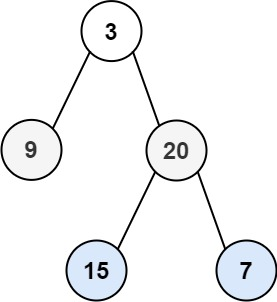``` Input: root = [3,9,20,null,null,15,7] Output: [,[9,20],[15,7]] ```

Example 2:

``` Input: root =  Output: [] ```

Example 3:

``` Input: root = [] Output: [] ```

2. Level Order – Solution

The following is a solution which demonstrates how to traverse binary tree level order.

This solution uses Breadth First Search to explore items at each level.

``` 2. Level Order - Solution C# // ============================================================================ // Author: Kenneth Perkins // Date: Oct 29, 2021 // Taken From: http://programmingnotes.org/ // File: Solution.cs // Description: Determines how to traverse a tree level order // ============================================================================ /** * Definition for a binary tree node. * public class TreeNode { * public int val; * public TreeNode left; * public TreeNode right; * public TreeNode(int val=0, TreeNode left=null, TreeNode right=null) { * this.val = val; * this.left = left; * this.right = right; * } * } */ public class Solution { public IList<IList<int>> LevelOrder(TreeNode root) { var result = new List<IList<int>>(); var queue = new Queue<TreeNode>(); if (root != null) { queue.Enqueue(root); } while (queue.Count > 0) { var level = new List<int>(); // Loop through items in the queue for (var itemCount = queue.Count; itemCount > 0; --itemCount) { var current = queue.Peek(); queue.Dequeue(); // Add children to the queue if they exist if (current.left != null) { queue.Enqueue(current.left); } if (current.right != null) { queue.Enqueue(current.right); } // Add current value to the level level.Add(current.val); } // Add items on this level to the result result.Add(level); } return result; } }// http://programmingnotes.org/ 1234567891011121314151617181920212223242526272829303132333435363738394041424344454647484950515253545556 // ============================================================================//    Author: Kenneth Perkins//    Date:   Oct 29, 2021//    Taken From: http://programmingnotes.org///    File:  Solution.cs//    Description: Determines how to traverse a tree level order// ============================================================================/** * Definition for a binary tree node. * public class TreeNode { *     public int val; *     public TreeNode left; *     public TreeNode right; *     public TreeNode(int val=0, TreeNode left=null, TreeNode right=null) { *         this.val = val; *         this.left = left; *         this.right = right; *     } * } */public class Solution {    public IList<IList<int>> LevelOrder(TreeNode root) {        var result = new List<IList<int>>();        var queue = new Queue<TreeNode>();         if (root != null) {            queue.Enqueue(root);        }         while (queue.Count > 0) {            var level = new List<int>();             // Loop through items in the queue            for (var itemCount = queue.Count; itemCount > 0; --itemCount) {                var current = queue.Peek();                queue.Dequeue();                 // Add children to the queue if they exist                if (current.left != null) {                    queue.Enqueue(current.left);                }                if (current.right != null) {                    queue.Enqueue(current.right);                }                 // Add current value to the level                level.Add(current.val);            }             // Add items on this level to the result            result.Add(level);        }         return result;    }}// http://programmingnotes.org/ ```

QUICK NOTES:
The highlighted lines are sections of interest to look out for.

The code is heavily commented, so no further insight is necessary. If you have any questions, feel free to leave a comment below.

Once compiled, you should get this as your output for the example cases:

``` [,[9,20],[15,7]] [] [] ```

## C# || How To Invert Binary Tree Using C#The following is a module with functions which demonstrates how to invert a binary tree using C#.

1. Invert Tree – Problem Statement

Given the root of a binary tree, invert the tree, and return its root.

Example 1: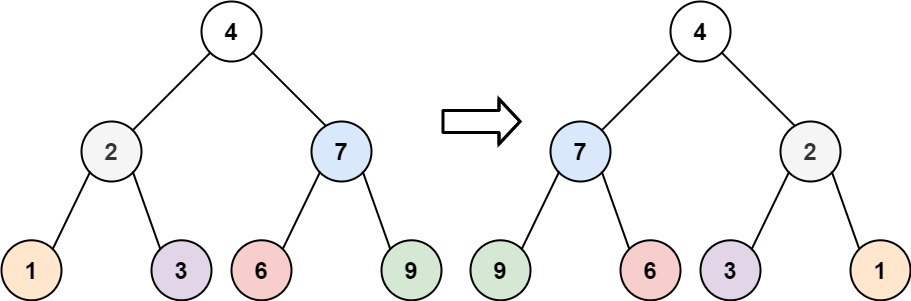``` Input: root = [4,2,7,1,3,6,9] Output: [4,7,2,9,6,3,1] ```

Example 2: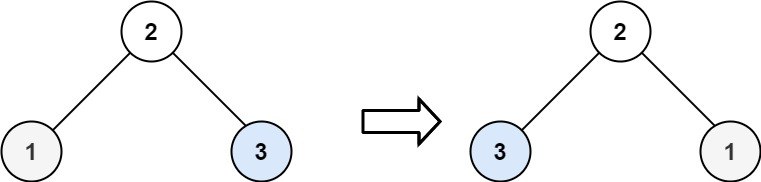``` Input: root = [2,1,3] Output: [2,3,1] ```

Example 3:

``` Input: root = [] Output: [] ```

2. Invert Tree – Solution

The following is a solution which demonstrates how to invert a binary tree.

An inverted Binary Tree is simply a Binary Tree whose left and right children are swapped.

This solution:

• Traverses the left subtree
• Traverses the right subtree
• When both trees have been traversed, swap left and right child subtrees

``` 2. Invert Tree - Solution C# // ============================================================================ // Author: Kenneth Perkins // Date: Oct 26, 2021 // Taken From: http://programmingnotes.org/ // File: Solution.cs // Description: Demonstrates how to invert a binary tree // ============================================================================ /** * Definition for a binary tree node. * public class TreeNode { * public int val; * public TreeNode left; * public TreeNode right; * public TreeNode(int val=0, TreeNode left=null, TreeNode right=null) { * this.val = val; * this.left = left; * this.right = right; * } * } */ public class Solution { public TreeNode InvertTree(TreeNode root) { Traverse(root); return root; } private void Traverse(TreeNode node) { if (node == null) { return; } // Keep traversing left and right nodes Traverse(node.left); Traverse(node.right); // Root has been visited, swap left and right child nodes var temp = node.left; node.left = node.right; node.right = temp; } }// http://programmingnotes.org/ 1234567891011121314151617181920212223242526272829303132333435363738394041 // ============================================================================//    Author: Kenneth Perkins//    Date:   Oct 26, 2021//    Taken From: http://programmingnotes.org///    File:  Solution.cs//    Description: Demonstrates how to invert a binary tree// ============================================================================/** * Definition for a binary tree node. * public class TreeNode { *     public int val; *     public TreeNode left; *     public TreeNode right; *     public TreeNode(int val=0, TreeNode left=null, TreeNode right=null) { *         this.val = val; *         this.left = left; *         this.right = right; *     } * } */public class Solution {    public TreeNode InvertTree(TreeNode root) {        Traverse(root);        return root;    }     private void Traverse(TreeNode node) {        if (node == null) {            return;        }         // Keep traversing left and right nodes        Traverse(node.left);        Traverse(node.right);         // Root has been visited, swap left and right child nodes        var temp = node.left;        node.left = node.right;        node.right = temp;    }}// http://programmingnotes.org/ ```

QUICK NOTES:
The highlighted lines are sections of interest to look out for.

The code is heavily commented, so no further insight is necessary. If you have any questions, feel free to leave a comment below.

Once compiled, you should get this as your output for the example cases:

``` [4,7,2,9,6,3,1] [2,3,1] [] ```

## C# || How To Determine Whether A Binary Tree Is A Symmetric Tree Using C#The following is a module with functions which demonstrates how to determine whether a binary tree is a symmetric tree using C#.

1. Is Symmetric – Problem Statement

Given the root of a binary tree, check whether it is a mirror of itself (i.e., symmetric around its center).

Example 1: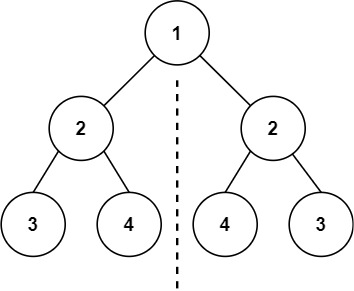``` Input: root = [1,2,2,3,4,4,3] Output: true ```

Example 2: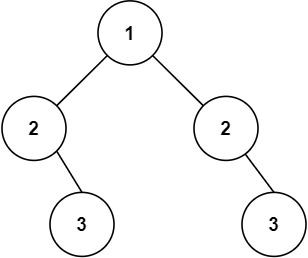``` Input: root = [1,2,2,null,3,null,3] Output: false ```

2. Is Symmetric – Solution

The following is a solution which demonstrates how to determine whether a binary tree is a symmetric tree.

For two trees to be mirror images, the following three conditions must be true:

``` • 1 - Their root node's key must be same • 2 - The left subtree of left tree and right subtree of right tree have to be mirror images • 3 - The right subtree of left tree and left subtree of right tree have to be mirror images ```

``` 2. Is Symmetric - Solution C# // ============================================================================ // Author: Kenneth Perkins // Date: Oct 20, 2021 // Taken From: http://programmingnotes.org/ // File: Solution.cs // Description: Determines whether a binary tree is a symmetric tree // ============================================================================ /** * Definition for a binary tree node. * public class TreeNode { * public int val; * public TreeNode left; * public TreeNode right; * public TreeNode(int val=0, TreeNode left=null, TreeNode right=null) { * this.val = val; * this.left = left; * this.right = right; * } * } */ public class Solution { public bool IsSymmetric(TreeNode root) { return IsMirror(root, root); } private bool IsMirror(TreeNode a, TreeNode b) { if (a == null && b == null) { return true; } if (a == null || b == null) { return false; } // 1. The two root nodes have the same value // 2. The left subtree of one root node is a mirror // reflection of the right subtree of the other root node // 3. The right subtree of one root node is a mirror // reflection of the left subtree of the other root node return a.val == b.val && IsMirror(a.left, b.right) && IsMirror(a.right, b.left); } }// http://programmingnotes.org/ 123456789101112131415161718192021222324252627282930313233343536373839404142 // ============================================================================//    Author: Kenneth Perkins//    Date:   Oct 20, 2021//    Taken From: http://programmingnotes.org///    File:  Solution.cs//    Description: Determines whether a binary tree is a symmetric tree// ============================================================================/** * Definition for a binary tree node. * public class TreeNode { *     public int val; *     public TreeNode left; *     public TreeNode right; *     public TreeNode(int val=0, TreeNode left=null, TreeNode right=null) { *         this.val = val; *         this.left = left; *         this.right = right; *     } * } */public class Solution {    public bool IsSymmetric(TreeNode root) {        return IsMirror(root, root);    }     private bool IsMirror(TreeNode a, TreeNode b) {        if (a == null && b == null) {            return true;        }        if (a == null || b == null) {            return false;        }        // 1. The two root nodes have the same value        // 2. The left subtree of one root node is a mirror        // reflection of the right subtree of the other root node        // 3. The right subtree of one root node is a mirror        // reflection of the left subtree of the other root node        return a.val == b.val &&            IsMirror(a.left, b.right) &&            IsMirror(a.right, b.left);    }}// http://programmingnotes.org/ ```

QUICK NOTES:
The highlighted lines are sections of interest to look out for.

The code is heavily commented, so no further insight is necessary. If you have any questions, feel free to leave a comment below.

Once compiled, you should get this as your output for the example cases:

``` true false ```

## C# || How To Traverse Bottom Up Binary Tree Level Order Using C#The following is a module with functions which demonstrates how to traverse bottom up binary tree level order using C#.

1. Level Order Bottom – Problem Statement

Given the root of a binary tree, return the bottom-up level order traversal of its nodes’ values. (i.e., from left to right, level by level from leaf to root).

Example 1:``` Input: root = [3,9,20,null,null,15,7] Output: [[15,7],[9,20],] ```

Example 2:

``` Input: root =  Output: [] ```

Example 3:

``` Input: root = [] Output: [] ```

2. Level Order Bottom – Solution

The following is a solution which demonstrates how to traverse bottom up binary tree level order.

The idea of this solution is to have a result list which keeps track of the items found on each level. A variable is also used to keep track of the maximum depth levels in the tree. The max depth level is used to insert node values into their appropriate result list slot.

``` 2. Level Order Bottom - Solution C# // ============================================================================ // Author: Kenneth Perkins // Date: Oct 20, 2021 // Taken From: http://programmingnotes.org/ // File: Solution.cs // Description: Determines how to traverse a tree bottom up level order // ============================================================================ /** * Definition for a binary tree node. * public class TreeNode { * public int val; * public TreeNode left; * public TreeNode right; * public TreeNode(int val=0, TreeNode left=null, TreeNode right=null) { * this.val = val; * this.left = left; * this.right = right; * } * } */ public class Solution { private List<IList<int>> result = new List<IList<int>>(); private int maxDepth = 0; public IList<IList<int>> LevelOrderBottom(TreeNode root) { Traverse(root, 0); return result; } private void Traverse(TreeNode node, int depth) { if (node == null) { return; } // Keep track of max depth level if (depth > maxDepth) { maxDepth = depth; } // Determine if new depth level should be added to the result list // Add the new level to the start of the result list if (result.Count == depth) { result.Insert(0, new List<int>()); } // Add the current node value to the corresponding result level index result[maxDepth - depth].Add(node.val); // Keep exploring left and right nodes Traverse(node.left, depth + 1); Traverse(node.right, depth + 1); } }// http://programmingnotes.org/ 1234567891011121314151617181920212223242526272829303132333435363738394041424344454647484950515253 // ============================================================================//    Author: Kenneth Perkins//    Date:   Oct 20, 2021//    Taken From: http://programmingnotes.org///    File:  Solution.cs//    Description: Determines how to traverse a tree bottom up level order// ============================================================================/** * Definition for a binary tree node. * public class TreeNode { *     public int val; *     public TreeNode left; *     public TreeNode right; *     public TreeNode(int val=0, TreeNode left=null, TreeNode right=null) { *         this.val = val; *         this.left = left; *         this.right = right; *     } * } */public class Solution {    private List<IList<int>> result = new List<IList<int>>();    private int maxDepth = 0;     public IList<IList<int>> LevelOrderBottom(TreeNode root) {        Traverse(root, 0);        return result;    }     private void Traverse(TreeNode node, int depth) {        if (node == null) {            return;        }         // Keep track of max depth level        if (depth > maxDepth) {            maxDepth = depth;        }         // Determine if new depth level should be added to the result list        // Add the new level to the start of the result list        if (result.Count == depth) {            result.Insert(0, new List<int>());        }         // Add the current node value to the corresponding result level index        result[maxDepth - depth].Add(node.val);         // Keep exploring left and right nodes        Traverse(node.left, depth + 1);        Traverse(node.right, depth + 1);    }}// http://programmingnotes.org/ ```

QUICK NOTES:
The highlighted lines are sections of interest to look out for.

The code is heavily commented, so no further insight is necessary. If you have any questions, feel free to leave a comment below.

Once compiled, you should get this as your output for the example cases:

``` [[15,7],[9,20],] [] [] ```